• 数据结构中 单链表 双链表 循环链表 c语言代码。
• 合肥工业大学数据结构试验一二（包括完整的试验要求、试验预习报告、最终试验报告） 试验内容： ...编写算法以构造带头结点的双循环链表。 编写算法以判断一个带头结点的双循环链表是否是对称的。
• int ListLen(LinkList L){ Node *p=L->next; int len=0; while(p!=NULL){ p=p->next; len++; } return len; }shell
• （1）单链表 编程实现一个单链表的建立/测长/打印。 #include #include typedef struct student {  int data;  struct student *next; }node; //建立单链表 node *creat() {  node *head,*p,*s;  int x,...
（1）单链表

编程实现一个单链表的建立/测长/打印。 #include <stdio.h>
#include <string.h>
typedef struct student
{
int data;
struct student *next;
}node;
//建立单链表
node *creat()
{
int x,cycle=1;

while(cycle)
{
scanf("%d",&x);
if(x!=0)
{
s=(node *)malloc(sizeof(node)); //为元素分配内存
s->data = x;
printf("\n%d",s->data);
p->next = s;                   //前一个元素的指针指向现在的元素
p=s;                           //现在的元素成为前一个元素
}
else cycle = 0;
}
p->next = NULL;                     //尾部指向NULL
}
//单链表测长
{
int n=0;
node *p;
while(p!=NULL)                       //链表结束
{
p=p->next;
n++;
}
return(n);
}
//单链表打印
{                                    //链表结束
node *p;int n;
printf("\nNow,These %d records are:\n",n);
while(p!=NULL)
{
printf("\n uuu %d ",p->data);
p = p->next;
}

}

如果删除的是中间节点，则把p2的next指向p1的next，同时free p1;
代码如下：
{
node *p1,*p2;
while(num!=p1->data && p1->next!=NULL) //挨着遍历,p2为找到的那个元素的前一个元素
{
p2=p1;
p1=p1->next;
}
if(num==p1->data)
{
{
free(p1);
}
else
{

p2->next = p1->next;//要删除的是中间节点（含尾节点）      free(p1);
}
}
else
printf("\n%d cound not be found",num);
}

编写程序实现单链表的插入。
如果插入在头节点以前，则p0的next指向p1,头节点指向p0;
如果插入中间节点，则先让p2的next指向p0,再让p0指向p1;
如果插入尾节点，则先让p1的next指向p0,再让p0指向空；
代码如下：
/*这里链表默认按从小到达排序*/
{
node *p0,*p1,*p2;
p0 = (node *)malloc(sizeof(node));
p0->data = num;
while(p0->data>p1->data && p1->next!=NULL)
{
p2=p1;
p1=p1->next;
}
if(p0->data<=p1->data)//判断不可少，因为可能到链表末尾了
{
{
p0->next = p1;
}
else
{
p2->next = p0;
p0->next = p1;
}
}
else
{
p1->next = p0;
p0->next = NULL;
}
}

编程实现单链表的排序 代码如下： /*单链表排序 双重循环 两两比较 每次找出剩下元素中最大的*/
{
node *p,*p2,*p3;
int n; int temp;
for(int i=1;i<n;i++)
{
for(int j=1;j<=n-i;j++)
{
if(p->data>p->next->data)   //两两比较，比后者大则互换
{
temp = p->data;
p->data = p->next->data;
p->next->data = temp;
}
p = p->next;
}
}
}

编程实现单链表的逆置。 代码如下： /*进行单链表逆置，首先要让p2的next指向p1 再让p1等于p2，p2等于p3,也就是说每次只调换一个箭头， 形成一个循环 */ node *reverse(node *head)
{
node *p1,*p2,*p3;
while(p2)
{
p3=p2->next;
p2->next = p1;
p1 = p2;
p2 = p3;
}
}

有一个C语言用来删除单链表的头元素的函数，请找出其中的问题并加以纠正。
{
}
正确答案如下：
(1)
{
node *p;
free(p);
}
(2)
{
node * p1;
free(p1);
}

给出一个单链表，不知道节点N的值，怎样只遍历一次就可以求出中间节点，写出算法。 想法很巧妙：设立两个指针，比如*p和*q。每次移动两个位置，即p=p->next->next,q每次移动一个位置，即q = q->next。当p到达最后一个节点时，q就是中间节点了。 void searchmid(node* head, node* mid)
{
{
temp = temp->next;
mid = temp;
}
}

（2）双链表

双链表与单链表类似，只是增加了一个前置链而已。 代码如下：
/*双链表建立仅仅比单链表建立多一个指向前一个元素的指针*/
#include <stdio.h>
#include <string.h>

typedef struct student
{
int data;
struct student *next;
struct student *pre;
}dnode;

dnode *creat()
{
int x,cycle = 1;
while(cycle)
{
scanf("%d",&x);
if(x!=0)
{
s=(dnode*)malloc(sizeof(dnode));
s->data=x;
printf("\n %d",s->data);
p->next = s;
s->pre  = p;
p = s;
}
else
cycle = 0;
}
p->next = NULL;

}
编程实现双链表删除/插入节点。
代码如下:

{
dnode *p1,*p2;
while(num!=p1->data && p1->next!=NULL)
p1 = p1->next;

if(num==p1->data)
{
{
free(p1);
}
else if(p1->next ==NULL)
{
p1->pre->next = NULL;
free(p1);
}
else
{
p1->next->pre = p1->pre;
p1->pre->next = p1->next;
//free(p1);
}
}
else
printf("\n%d cound not been found",num);
}
/*
双链表插入节点
*/
{
dnode *p0,*p1;
p0 = (dnode *)malloc(sizeof(dnode));
p0->data = num;
while(p0->data>p1->data && p1->next!=NULL)
p1= p1->next;
if(p0->data<=p1->data)
{
{
p0->next = p1;
p1->pre = p0;
}
else                        //删除中间
{
p1->pre->next = p0;
p0->next = p1;
p0->pre = p1->pre;
p1->pre = p0;
}
}
else                          //删除尾
{
p1->next = p0;
p0->pre = p1;
p0->next = NULL;
}
}

（3）循环链表
已知n个人（以编号1,2,3,...,n分别表示）围坐在一张圆桌周围。从编号为k的人开始报数，数到m的那个人出列；他的下一个人又从1开始报数，数到m的那个人又出列；依次规律重复下去，直到圆桌周围的人全部出列。试用c++编程实现。
先给一个简单的版本，即没有k，直接从1开始的：
//初始位置从1开始
#include <stdio.h>
#include <stdlib.h>
{
struct node
{
int data;
struct node *next;
}NODE:
*pri;

for(int i=2;i<=n;i++)
{
p=(node *)malloc(sizeof(struct node));
p->data = i;
p->next = NULL;
q->next = p;
q = q->next;
}

while(peopel<n)
{
pri = pri->next;
p = p->next;
{
printf("%d",p->data);
node *temp;
temp = p;
pri->next = p->next;
p = p->next;
free(temp);
people++；
}
}
printf("\n");
return 0;
}

int main()
{
int n,m;
printf("请输入人数和密码")；
while(scanf("%d%d",&n,&m)!=EOF)
{
if(m<0||n<0)
{
printf("输入错误，请重新输入\n");
continue;
}
}
system("pause");
return 0;
}

再给一个版本：大同小异，不过一开始把指针移动到K处的位置，两个版本结合理解
//初始位置从K开始
#include <stdlib.h>
#include <stdio.h>
#define ERROR 0
typedef struct LNode{
int data;

void JOSEPHUS(int n,int k, int m)
//n为总人数，k为第一个开始报数的人，m为出列者喊道的数
{
/*p为当前节点，r为辅助节点，指向p的前驱节点，list为头节点*/
/*建立循环链表*/

curr = p;

for(int i=1;i<n;i++)   //与xuanhuanlianbiao1的区别在于始终保持了尾节点指向了头节点，前者则是尾节点指向NULL，最后出循环后再指向头节点
{
t->data = i;
curr = t;
}
/*把当前指针移动到第一个报数的人*/
r = curr;
while(k--)
{
}
while(n--)
{
printf("%d->",p->data);
free(p);
}
}

main()
{
JOSEPHUS(15,5,8);
}

附录：单链表 双链表 循环链表 完整.c文件下载(http://www.t00y.com/file/31447179)。 
展开全文数据结构
• 这个资料实现的是循环单链表-双链表，为初学者提供源码
• 新手，最近在学c#，请问有谁知道关于链表的视频，包括单链表，单循环链表双循环链表等等，从链表最基础的开始讲的视频，里面介绍使用链表最基础的代码，谢了谢了！c#
• 单链表 双向链表 基于Java的单链表双向链表实现
一、单链表

单链表是一种链式存取的数据结构，用一组地址任意的存储单元存放线性表中的数据元素。链表中的数据是以结点来表示的，每个结点的构成：元素(数据元素的映象) + 指针(指示后继元素存储位置)，元素就是存储数据的存储单元，指针就是连接每个结点的地址数据。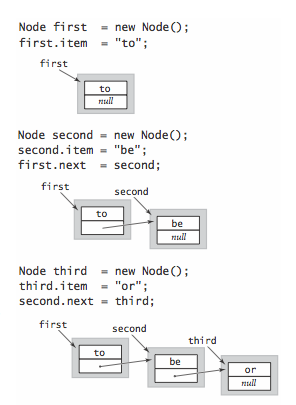在开头插入。在链接列表中插入新节点的最简单位置是开始。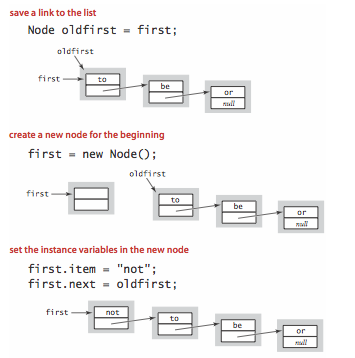从头开始删除。删除链表中的第一个节点也很容易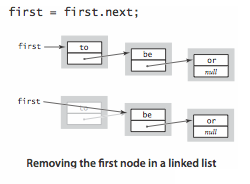在末尾插入。要在链接列表的末尾插入一个节点，我们维护一个指向列表中最后一个节点的链接。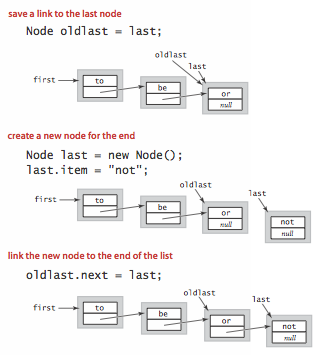/**
* 单链表
*/
Node HeadNode = new Node(0, "", "");//创建链表时头结点便已经创建完成在

//增加节点
while (temp.next != null) temp = temp.next;
temp.next = node;
}

/**
* 按no的顺序添加节点
*/
while (temp.next != null) {//如果没有大小匹配的，就放在末尾
if (temp.no < node.no && temp.next.no > node.no) break;
}
if (temp.next == null) {
temp.next = node;
} else {
node.next = temp.next;
temp.next = node;
}
}

/**
* 根据no修改信息
*/
public void updateNodeByNo(Node newNode) {
while (true) {
if (temp.no == newNode.no) {
temp.name = newNode.name;
temp.nickName = newNode.nickName;
break;
}
//最后一个节点
if (temp.next == null) throw new RuntimeException("no错误");
temp = temp.next;
}
}

/**
* 删除节点
*/
public void deletNodeByNo(int no) {
if (temp.next == null) {
System.out.println("链表为空");
return;
}
Node pre = temp;//前驱指针
temp = temp.next;
while (true) {
if (temp.no == no) {
pre.next = temp.next;
break;
}
if (temp.next == null) break;
pre = temp;
temp = temp.next;
}
}

/**
* 遍历当前链表
*/
System.out.println("链表为空");
return;
}
while (true) {
System.out.println(temp);
if (temp.next == null) break;
temp = temp.next;
}
}
}

节点类
/**
* 节点类
*/
class Node {
public int no;//编号
public String name;
public String nickName;
public Node next;//指向下一个节点

//节点的构造方法
public Node(int no, String name, String nickName) {
this.no = no;
this.name = name;
this.nickName = nickName;
this.next = null;
}

@Override
public String toString() {
return new StringJoiner(", ", Node.class.getSimpleName() + "[", "]")
.toString();
}
}

二、双向链表

双向链表也叫双链表，是链表的一种，它的每个数据结点中都有两个指针，分别指向直接后继和直接前驱。所以，从双向链表中的任意一个结点开始，都可以很方便地访问它的前驱结点和后继结点。一般我们都构造双向循环链表。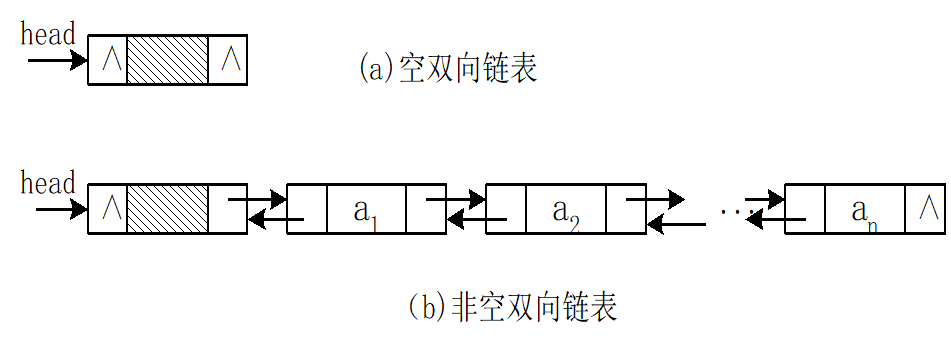/**
* 链表类
*/

private DoubleNode FirstNode = new DoubleNode(0, "");//创建链表时先初始化一个头结点

/**
* 最后一个节点后
*
* @param node
*/
DoubleNode temp = FirstNode;
while (temp.getNext() != null) {
temp = temp.getNext();
}
temp.setNext(node);
node.setPre(temp);
}

/**
* 按顺序添加节点
*/
DoubleNode temp = FirstNode;
while (true) {
if (temp.getNo() == node.getNo()) {
System.out.println("节点已经存在");
return;
}
if (temp.getNo() > node.getNo()) {//中间插入
temp.getPre().setNext(node);
node.setPre(temp.getPre());
node.setNext(temp);
temp.setPre(node);
break;
}
if (temp.getNext() == null) {//最后一个节点
temp.setNext(node);
node.setPre(temp);
break;
}
temp = temp.getNext();
}
}

/**
* 遍历链表
*/
if (FirstNode.getNext() == null) {
System.out.println("链表为空");
return;
}
DoubleNode temp = FirstNode.getNext();
while (true) {
System.out.println(temp);
if (temp.getNext() == null) break;
temp = temp.getNext();
}
}

/**
* 删除节点
*/
public void deleteNode(int no) {
if (FirstNode.getNext() == null) {
System.out.println("链表为空");
return;
}
DoubleNode temp = FirstNode.getNext();
while (true) {
if (temp.getNo() == no) {
temp.getPre().setNext(temp.getNext());
temp.getNext().setPre(temp.getPre());
}
if (temp.getNext() == null) break;
temp = temp.getNext();
}
}

/**
* 修改节点信息
*/
public void updateNode(DoubleNode newNode) {
if (FirstNode.getNext() == null) {
System.out.println("链表为空");
return;
}
DoubleNode temp = FirstNode.getNext();
while (true) {
if (temp.getNo() == newNode.getNo()) {
temp.setName(newNode.getName());
break;
}
if (temp.getNext() == null) {
System.out.println("没有相关节点");
break;
}
temp = temp.getNext();
}
}
}

节点类
/**
* 节点类
*/
class DoubleNode {
private int no;
private String name;
private DoubleNode next;//指向后一个节点
private DoubleNode pre;//指向前一个节点

public DoubleNode(int no, String name) {
this.no = no;
this.name = name;
}

public int getNo() {
return no;
}

public void setNo(int no) {
this.no = no;
}

public String getName() {
return name;
}

public void setName(String name) {
this.name = name;
}

public DoubleNode getNext() {
return next;
}

public void setNext(DoubleNode next) {
this.next = next;
}

public DoubleNode getPre() {
return pre;
}

public void setPre(DoubleNode pre) {
this.pre = pre;
}

@Override
public String toString() {
return new StringJoiner(", ", DoubleNode.class.getSimpleName() + "[", "]")
.toString();
}
}

展开全文java 数据结构
• 目录 1 单链表的应用实例 ...1.4.5 合并两个有序的单链表，合并之后的链表依然有序 2 双向链表 2.1 添加 2.2 删除 1) 链表是以节点的方式来存储, 是链式存储 2) 每个节点包含 data 域， next 域：指
目录
1 单链表的应用实例
1.1 添加
1.1.1 直接添加到尾部
1.1.2 插入到指定位置
1.2 修改节点
1.3 删除节点
1.4 应用
1.4.1 求单链表有效节点个数
1.4.2 查找单链表中的倒数第k个节点
1.4.3 单链表的反转
1.4.4 从头到尾打印单链表
1.4.5 合并两个有序的单链表，合并之后的链表依然有序
2 双向链表
2.1 添加
2.2 删除

1) 链表是以节点的方式来存储, 是链式存储 2) 每个节点包含 data 域， next 域：指向下一个节点. 3) 发现 链表的各个节点不一定是连续存储. 4) 链表分 带头节点的链表和 没有头节点的链表，根据实际的需求来确定
1 单链表的应用实例
1.1 添加
1.1.1 直接添加到尾部
思路，当不考虑编号顺序时 1. 找到当前链表的最后节点 2. 将最后这个节点的next 指向 新的节点
		//因为head节点不能动，因此我们需要一个辅助遍历 temp
//遍历链表，找到最后
while(true) {
//找到链表的最后
if(temp.next == null) {//
break;
}
//如果没有找到最后, 将将temp后移
temp = temp.next;
}
//当退出while循环时，temp就指向了链表的最后
//将最后这个节点的next 指向 新的节点
temp.next = heroNode;
1.1.2 插入到指定位置
找到要插入的位置，通过辅助变量遍历新的节点.next=temp（辅助变量）.next将temp.next=新的节点
		//因为头节点不能动，因此我们仍然通过一个辅助指针(变量)来帮助找到添加的位置
//因为单链表，因为我们找的temp 是位于 添加位置的前一个节点，否则插入不了
boolean flag = false; // flag标志添加的编号是否存在，默认为false
while(true) {
if(temp.next == null) {//说明temp已经在链表的最后
break; //
}
if(temp.next.no > heroNode.no) { //位置找到，就在temp的后面插入
break;
} else if (temp.next.no == heroNode.no) {//说明希望添加的heroNode的编号已然存在

flag = true; //说明编号存在
break;
}
temp = temp.next; //后移，遍历当前链表
}
//判断flag 的值
if(flag) { //不能添加，说明编号存在
System.out.printf("准备插入的英雄的编号 %d 已经存在了, 不能加入\n", heroNode.no);
} else {
//插入到链表中, temp的后面
heroNode.next = temp.next;
temp.next = heroNode;
}
1.2 修改节点
通过遍历找到该节点，修改节点信息
1.3 删除节点
先找到需要删除的节点的前一个节点temptemp.next=temp.next.next被删除的节点将不会有其他引用指向，会被垃圾回收机制回收
1.4 应用
1.4.1 求单链表有效节点个数
	//方法：获取到单链表的节点的个数(如果是带头结点的链表，需求不统计头节点)
/**
*
* @return 返回的就是有效节点的个数
*/
public static int getLength(HeroNode head) {
return 0;
}
int length = 0;
//定义一个辅助的变量, 这里我们没有统计头节点
while(cur != null) {
length++;
cur = cur.next; //遍历
}
return length;
}
1.4.2 查找单链表中的倒数第k个节点
思路
编写一个方法，接收 head 节点，同时接收一个 index index 表示是倒数第 index 个节点先把链表从头到尾遍历，得到链表的总的长度 getLength 得到 size 后，我们从链表的第一个开始遍历 (size-index)个，就可以得到 如果找到了，则返回该节点，否则返回 nulll
	public static HeroNode findLastIndexNode(HeroNode head, int index) {
//判断如果链表为空，返回null
return null;//没有找到
}
//第一个遍历得到链表的长度(节点个数)
//第二次遍历  size-index 位置，就是我们倒数的第K个节点
//先做一个index的校验
if(index <=0 || index > size) {
return null;
}
//定义给辅助变量， for 循环定位到倒数的index
HeroNode cur = head.next; //3 // 3 - 1 = 2
for(int i =0; i< size - index; i++) {
cur = cur.next;
}
return cur;

}
1.4.3 单链表的反转
定义一个新的链表
从头到尾遍历链表，没遍历一个节点就把该节点取出来，并采用头插法插入新链表
	public static void reversetList(HeroNode head) {
//如果当前链表为空，或者只有一个节点，无需反转，直接返回
return ;
}

//定义一个辅助的指针(变量)，帮助我们遍历原来的链表
HeroNode next = null;// 指向当前节点[cur]的下一个节点
HeroNode reverseHead = new HeroNode(0, "", "");
//动脑筋
while(cur != null) {
next = cur.next;//先暂时保存当前节点的下一个节点，因为后面需要使用
cur = next;//让cur后移
}
}
1.4.4 从头到尾打印单链表
方法一：倒置链表然后打印
缺陷：改变了链表的结构
方法二：遍历链表，把每一个节点存入栈中，然后出栈
	public static void reversePrint(HeroNode head) {
return;//空链表，不能打印
}
//创建要给一个栈，将各个节点压入栈
Stack<HeroNode> stack = new Stack<HeroNode>();
//将链表的所有节点压入栈
while(cur != null) {
stack.push(cur);
cur = cur.next; //cur后移，这样就可以压入下一个节点
}
//将栈中的节点进行打印,pop 出栈
while (stack.size() > 0) {
System.out.println(stack.pop()); //stack的特点是先进后出
}
}
1.4.5 合并两个有序的单链表，合并之后的链表依然有序
同1.4.3单链表的倒置，新建一个链表，遍历两个链表，比较大小，在让原来的头结点指向新链表
2 双向链表
2.1 添加
先找到双向链表的最后这个节点temp.next = newHeroNodenewHeroNode.pre = temp;
2.2 删除
因为是双向链表，因此，我们可以实现自我删除某个节点直接找到要删除的这个节点，比如 temptemp.pre.next = temp.nexttemp.next.pre = temp.pre;


展开全文• 最近在面试过程中总被问到链表的问题,因此这里把自己实现的链表操作上传给大家共享!包括单链表,双链表,循环单链表,循环双链表的(可重复)插入和(可重复)删除的完整实现,由c及Code::Blacks实现.
• 循环链表单链表中，从一已知结点出发，只能访问到该结点及其后续结点，无法找到该结点之前的其它结点。而在单循环链表中，从任一结点出发都可访问到表中所有结点，这一优点使某些运算在单循环链表上易于实现。 ...
单链表：拥有指向下一节点的指针。查找元素需要从头结点遍历
双链表：拥有指向上一节点和下一节点的指针。相对于单链表，在找当前节点的前一节点时比较方便，占用空间比单链表大。
单循环链表：单链表中，从一已知结点出发，只能访问到该结点及其后续结点，无法找到该结点之前的其它结点。而在单循环链表中，从任一结点出发都可访问到表中所有结点，这一优点使某些运算在单循环链表上易于实现。
双循环链表：可以从任何结点开始任意向前向后双向访问。
单链表和单循环链表：只能在当前结点后插入和删除。
双链表：可以在当前结点前面或者后面插入，可以删除前趋和后继（包括结点自己）。
存储：单链表和单循环链表存储密度大于双链表。
哈希表：在哈希表中进行添加，删除，查找等操作，性能十分之高，不考虑哈希冲突的情况下，仅需一次定位即可完成，时间复杂度为O(1)。我们要新增或查找某个元素，我们通过把当前元素的关键字 通过某个函数映射到数组中的某个位置，通过数组下标一次定位就可完成操作。
存储位置 = f(关键字)
其中，这个函数f一般称为哈希函数，这个函数的设计好坏会直接影响到哈希表的优劣。例如：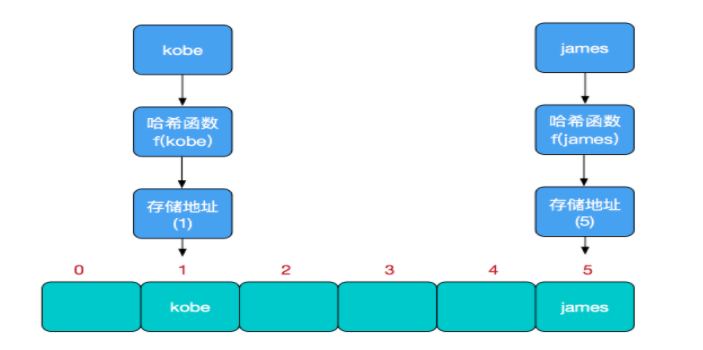当我们对某个元素进行哈希运算，得到一个存储地址，然后要进行插入的时候，发现已经被其他元素占用了，其实这就是所谓的哈希冲突，也叫哈希碰撞。数组是一块连续的固定长度的内存空间，再好的哈希函数也不能保证得到的存储地址绝对不发生冲突。哈希冲突的解决方案有多种:开放定址法（发生冲突，继续寻找下一块未被占用的存储地址），再散列函数法，链地址法，而HashMap即是采用了链地址法，也就是数组+链表的方式，
hashmap：简单来说，HashMap由数组+链表组成的，数组是HashMap的主体，链表则是主要为了解决哈希冲突而存在的，如果定位到的数组位置不含链表（当前entry的next指向null）,那么对于查找，添加等操作很快，仅需一次寻址即可；如果定位到的数组包含链表，对于添加操作，其时间复杂度为O(n)，首先遍历链表，存在即覆盖，否则新增；对于查找操作来讲，仍需遍历链表，然后通过key对象的equals方法逐一比对查找。所以，性能考虑，HashMap中的链表出现越少，性能才会越好。
展开全文• 单链表实现双向循环链表单向链表存在一个弊端就是，当需要获取某个结点p的前驱时，需要从头指针开始遍历链表，获得“前驱”的执行时间为O(n)，为了克服单向链表的这种缺点，可以利用双向链表。在双向链表中有两个...
• 文档包括带表头单链表的实现，双链表的操作，双链表的冒泡排序，不带表头的单链表，双向链表、链表节点的排序
• ## 单链表、双向链表、循环链表

千次阅读 多人点赞 2019-07-26 14:26:09
学习三种常见的链表结构，他们分别是：单链表、双向链表和循环链表单链表 单链表有两个较特殊节点，头结点和尾节点。头结点用来记录链表的基地址，可以用来遍历整条链表。尾结点的指针不是指向下一个节点而是指向...数据结构 单向链表 双向链表
• 文章目录前言单链表单循环链表双链表循环链表错误纠正说明时间复杂度比较关于头结点 前言 博主最近在复习算法与数据结构，由于平时主力语言是Python，所以找了个用Python讲解数据结构的视频看了下，链接为：...Python
• 单链表结点类Node声明如下，成员变量data表示结点的数据域，保存数据元素，数据类型为T，next表示结点的指针域，保存后继结点的地址。文件名为Node.h  template  class Node  {  public;  T data;  ...数据结构
• 1.单链表的基本操作（C语言） 创建头节点 Linklist *Creat(Linklist *L) { L = (Linklist *)malloc(sizeof(Linklist)); if (L == NULL) return NULL; /*内存分配不成功,返回空指针*/ L -> next = NULL; ...c语言
• 双链表 双向循环链表 单链表 实现代码如下： #include &lt;stdio.h&gt; #include &lt;stdlib.h&gt; #include &lt;assert.h&gt; typedef int DataType; typede...C语言 数据结构
• 链表不仅作为链式存储的一种实现方式，还表达了计算机不连续（离散）的存储思想。数据结构 链表
• 链表介绍 不带头结点的单向链表 带头结点的单向链表 循环链表 双向循环链表双向链表 时间复杂度
• 文章目录循环链表循环单链表循环双链表静态链表说明 循环链表循环链表一般包括循环单链表和循环双链表，如下图所示： 循环单链表   循环单链表单链表的区别在于，表中最后一个结点的指针不是NULL，而改为...
• 1.头指针和头结点 头指针 指向第一个模块。 头结点 在链表的第一个结点之前附设一个结点,这个结点可以不存储信息，也可以存储链表的长度等。 2.单链表 单链表，只在尾部有一个指针，指向下一个...双向循环链表 首尾数据结构
• 单向链表（单链表） 单向链表，它包含两个域，一个信息域和一个指针域。这个链接指向表中的下一个节点，而最后一个节点则 指向一个空值NULL。 单向链表只可向一个方向遍历。...双向链表,（双链表） 双向链表中不链表 指针
• 对于单链表、单循环链表和双向链表，如果仅仅知道一个指向链表中某个节点链表的指针P，能否将P所指结点的数据元素与其确实存在的直接前驱？请对每一中链表作出判断，若可以，写出程序段；否则说明理由。 单链表...
• 循环链表前言一、循环单链表的初始化二、判断是否为空或表尾结点三、循环双链表的初始化四、循环双链表的插入与删除 前言 对于循环单链表而言，在进行插入和删除时，可以让指针指向尾部，对于表头(通过尾部找到头部...数据结构数据结构...